Question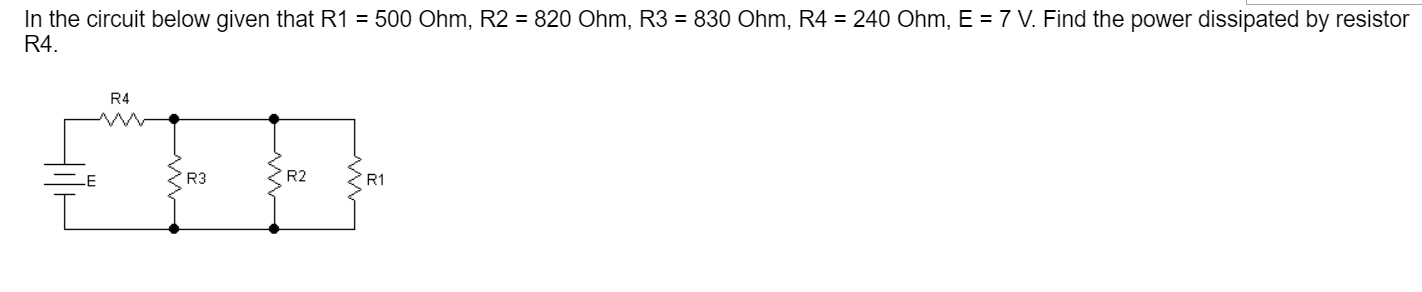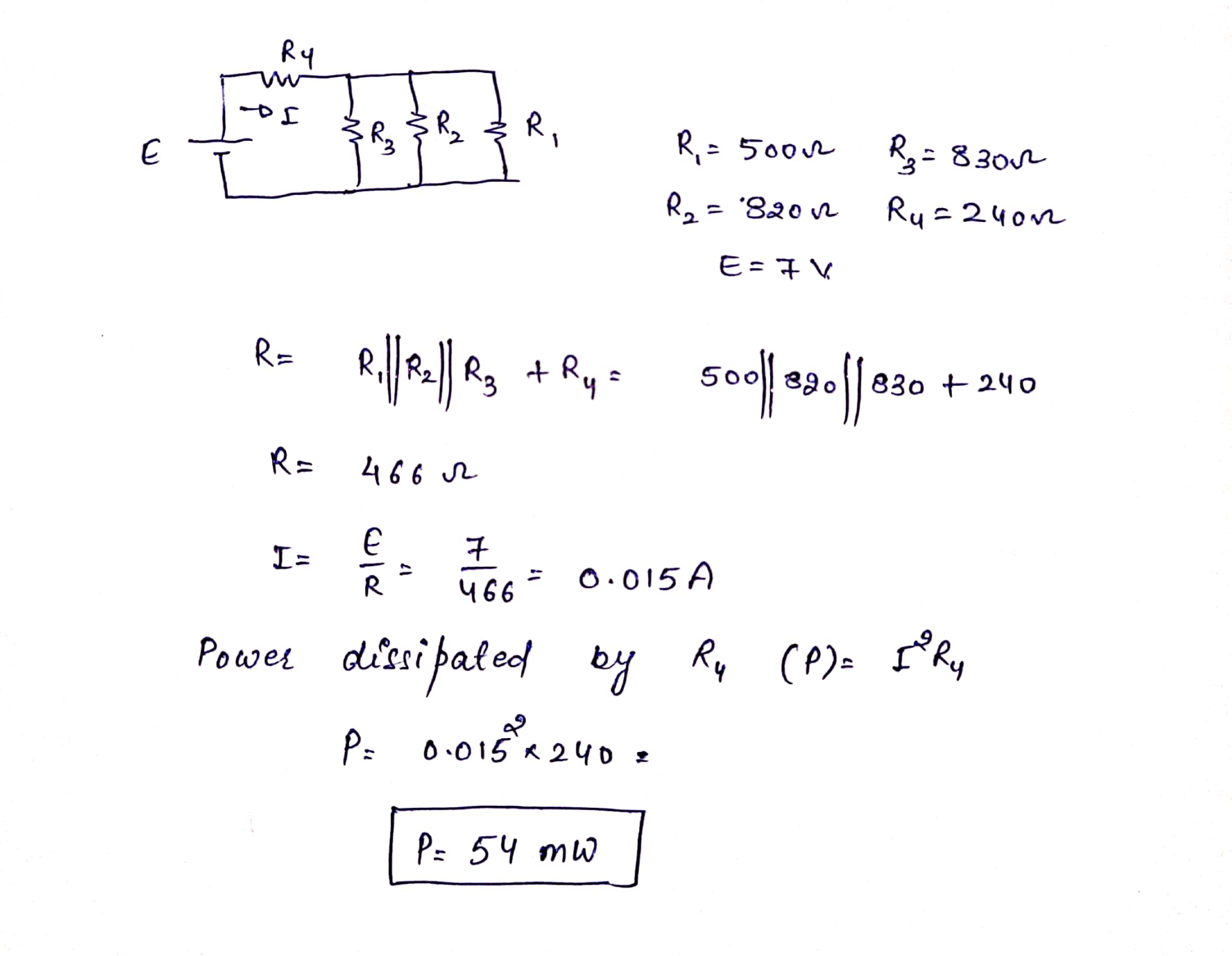#### Earn Coins

Coins can be redeemed for fabulous gifts.

Similar Homework Help Questions
• ### Consider the circuit shown below: R1 = 600 ohm R2 = 480 ohm R3 = 240...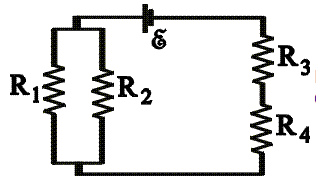Consider the circuit shown below: R1 = 600 ohm R2 = 480 ohm R3 = 240 ohm R4 = 210 ohm ε = 4.5 V a)Calculate the potential difference across R4. b)Calculate the power dissipated in R3. AA CN.

• ### R2 R1 R3 R4 E In the following circuit, R1=0.8 4 Ohm, R2-2' 4 Ohm, R3-3'...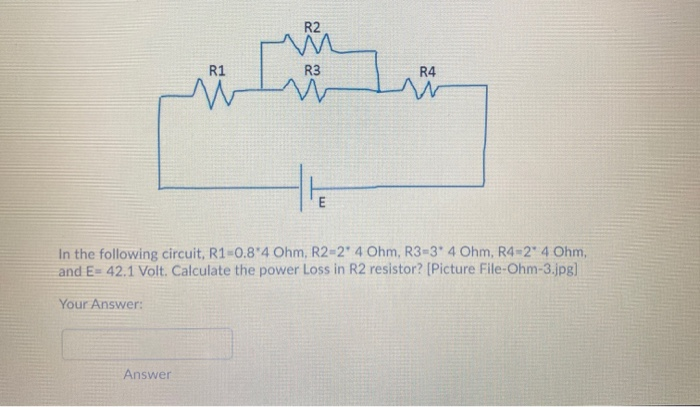R2 R1 R3 R4 E In the following circuit, R1=0.8 4 Ohm, R2-2' 4 Ohm, R3-3' 4 Ohm, R4-2° 4 Ohm, and E= 42.1 Volt. Calculate the power Loss in R2 resistor? [Picture File-Ohm-3.jpg] Your Answer: Answer

• ### R2 w R3 M R1 R4 » E In the following circuit, R1=0.8*4 Ohm, R2=2* 4...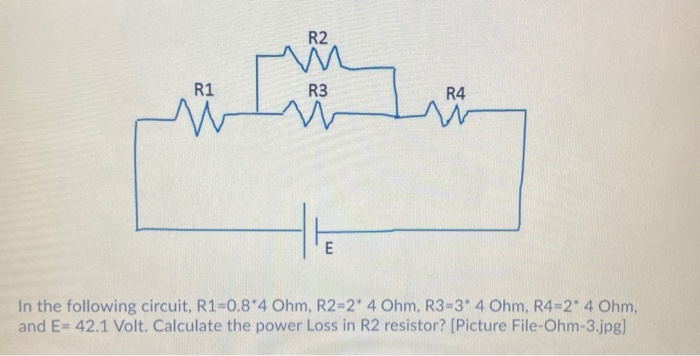R2 w R3 M R1 R4 » E In the following circuit, R1=0.8*4 Ohm, R2=2* 4 Ohm, R3-3* 4 Ohm, R4=2* 4 Ohm, and E= 42.1 Volt. Calculate the power Loss in R2 resistor? [Picture File-Ohm-3.jpg]

• ### R2 R1 R3 R5 M R4 HE In the following circuit, R1=3 Ohm, R2=4* 4.9 Ohm,...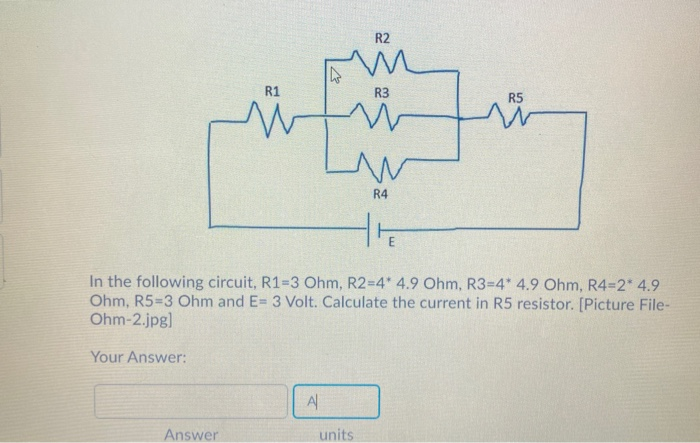R2 R1 R3 R5 M R4 HE In the following circuit, R1=3 Ohm, R2=4* 4.9 Ohm, R3=4* 4.9 Ohm, R4=2* 4.9 Ohm, R5=3 Ohm and E= 3 Volt. Calculate the current in R5 resistor. [Picture File- Ohm-2.jpg) Your Answer: AL Answer units

• ### For the following circuit, assume that R1 = 1 ohm, R2 = 2 ohms, R3 =...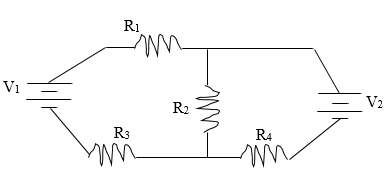For the following circuit, assume that R1 = 1 ohm, R2 = 2 ohms, R3 = 8 ohms, and R4 = 4 ohms. Also assume that V1 = 50 volts and V2 = 100 volts. Find the current through each of the resistors and the power dissipated through all of the resistors. (Hint: You’ll need to use Kirchoff’s Loop and Junction Rule) Vi R2 R3 R4 NV

• ### In the circuit, V1=129 V, V2=72 V, I0=2 A, R1=7 Ohm, R2=46 Ohm, R3=19 Ohm, R4=19...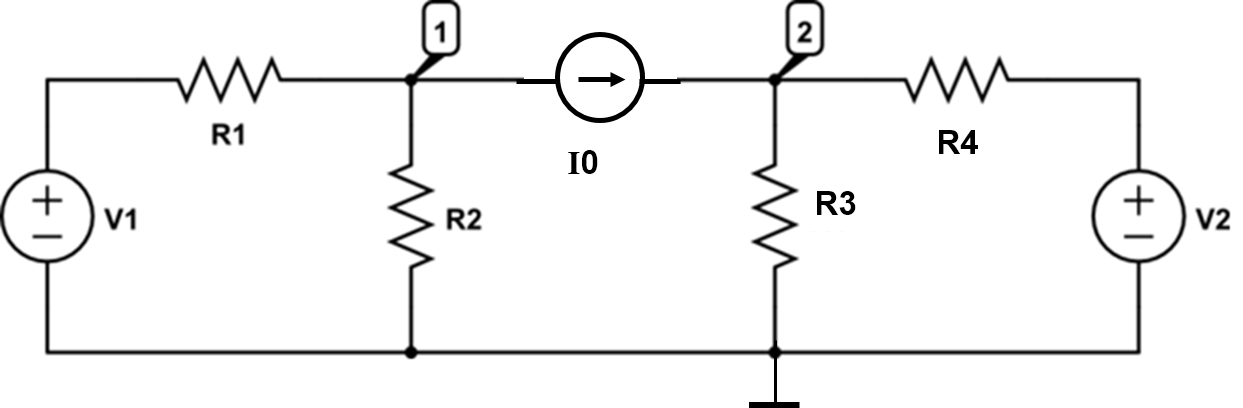In the circuit, V1=129 V, V2=72 V, I0=2 A, R1=7 Ohm, R2=46 Ohm, R3=19 Ohm, R4=19 Ohm. Solve the circuit using nodal analysis and answer the questions below. Find the current through R1 flowing in the direction from left to right. (Unit: A) Find the current through R2 flowing downwards. (Unit: A) Find the current through R3 flowing downwards. (Unit: A) Find the current thrrough R4 flowing from left to right. (Unit: A) R1 R4

• ### In the following circuit, R1=1 Ohm, R2=4* 4.6 Ohm, R3=4* 4.6 Ohm, R4=2* 4.6 Ohm, R5=1...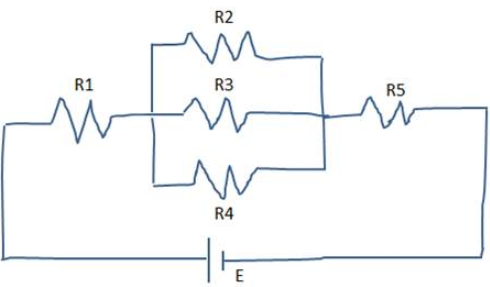In the following circuit, R1=1 Ohm, R2=4* 4.6 Ohm, R3=4* 4.6 Ohm, R4=2* 4.6 Ohm, R5=1 Ohm and E= 1 Volt. Calculate the current in R5 resistor. [Picture File-Ohm-2.jpg] R2 R3 R1 R5 R4 E

• ### Question 12 (1 point) In the following circuit, R1-0.8*12 Ohm, R2-2 12 Ohm, R3-3 12 Ohm,...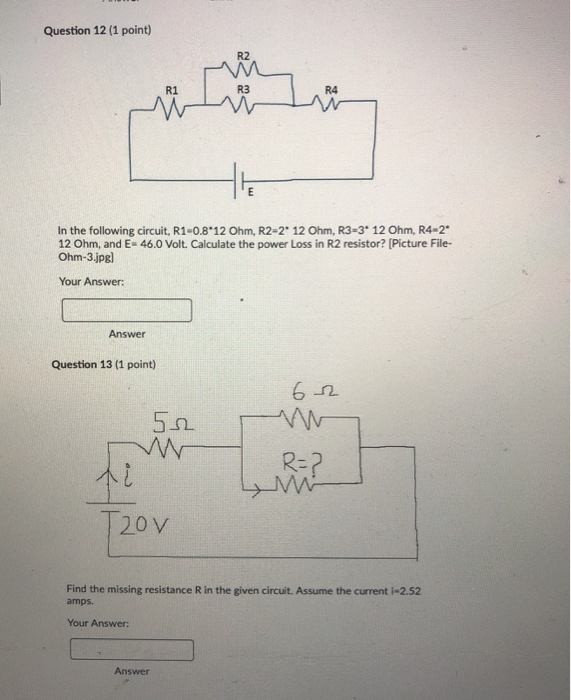Question 12 (1 point) In the following circuit, R1-0.8*12 Ohm, R2-2 12 Ohm, R3-3 12 Ohm, R4-2* 12 Ohm, and E-46.0 Volt. Calculate the power Loss in R2 resistor? (Picture File- Ohm-3.jpg) Your Answer: Answer Question 13 (1 point) 62 be R=2 20v Find the missing resistance R in the given circuit. Assume the current i-2.52 amps. Your Answer: Answer

• ### Question 8 (1 point) R2 W R3 In the following circuit, R1=14 Ohm, R2=4" 1.4 Ohm,...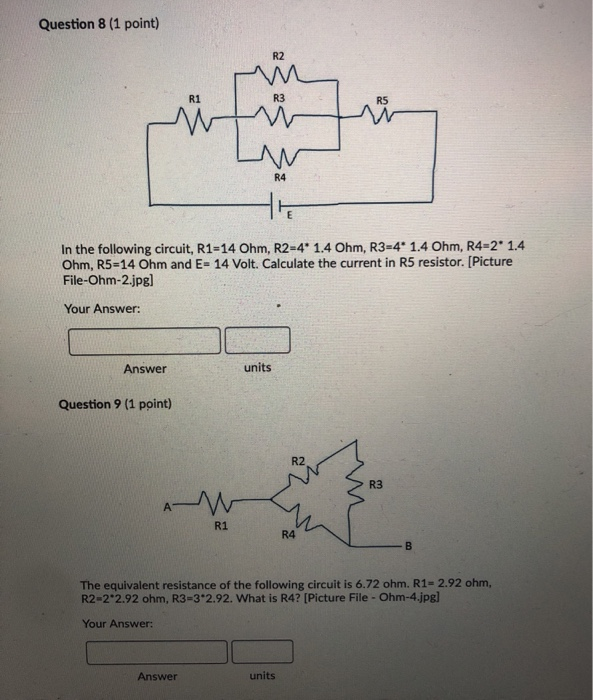Question 8 (1 point) R2 W R3 In the following circuit, R1=14 Ohm, R2=4" 1.4 Ohm, R3=41.4 Ohm, R4-2 1.4 Ohm, R5-14 Ohm and E= 14 Volt. Calculate the current in R5 resistor. (Picture File-Ohm-2.jpg] Your Answer: Answer units Question 9 (1 point) The equivalent resistance of the following circuit is 6.72 ohm. R1= 2.92 ohm, R2-2 2.92 ohm, R3=3*2.92. What is R4? [Picture File - Ohm-4.jpg] Your Answer: Answer units

• ### R1 R2 R4 Is R3 Given that Vs is 18 V, Is is 6 A, R1...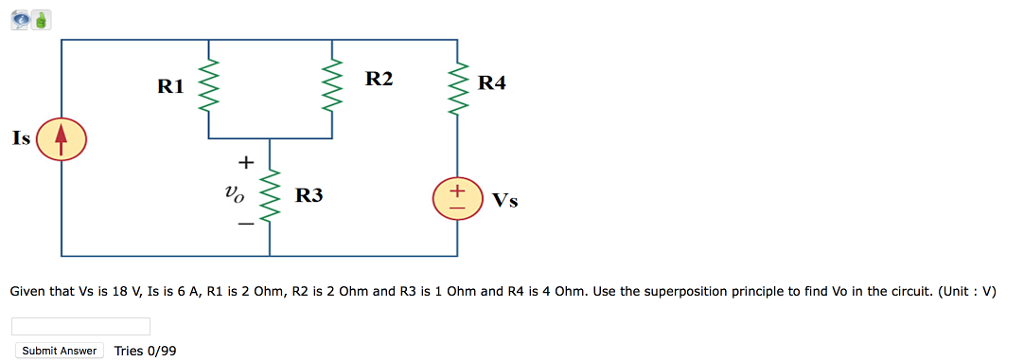R1 R2 R4 Is R3 Given that Vs is 18 V, Is is 6 A, R1 is 2 Ohm, R2 is 2 Ohm and R3 is 1 Ohm and R4 is 4 Ohm. Use the superposition principle to find Vo in the circuit. (Unit V) Submit Answer Tries 0/99